# Arithmetic and Geometric Progressions

Arithmetic and Geometric Progressions

In this mini-lesson, we will explore the world of arithmetic progression and geometric progression in math. You will get to learn about the arithmetic progression formula, geometric progression formula, sum of arithmetic progression, geometric progression sum, and other interesting facts around the topic. You can also check out the playful calculators to know more about the lesson and try your hand at solving a few interesting practice questions at the end of the page.

Let's start by answering a simple question. Have you ever observed patterns in your day-to-day life?

For example, Olivia and Betty are walking along a street to visit their friend, Hailey.

As they walk, they observe a pattern of house numbers on the street.

These numbers are in ascending order.Here, these house numbers form a sequence.

Let's go ahead and learn about two types of sequences: arithmetic progression and geometric progression.

## What Is Definition And General Notations of Arithmetic Progression and Geometric Progression?

### Definition of Arithmetic Progression

An arithmetic progression is a sequence of numbers in which each successive term is a sum of its preceding term and a fixed number.

This fixed number is called a common difference.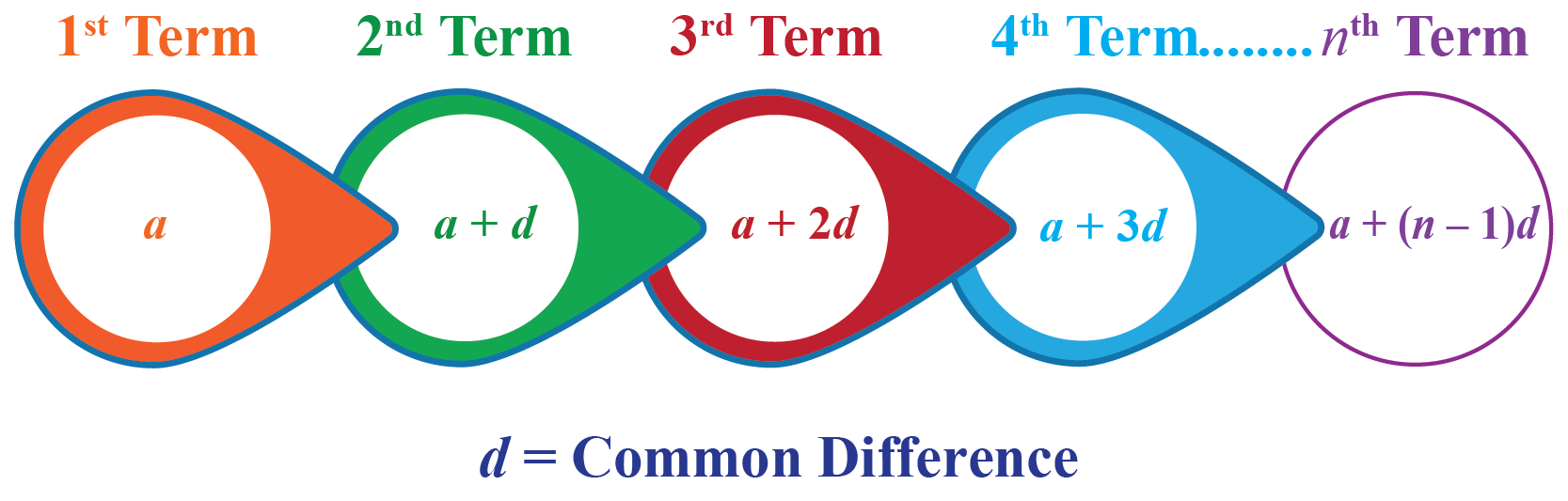### Definition of Geometric Progression

A geometric progression is a sequence where every term bears a constant ratio to its preceding term.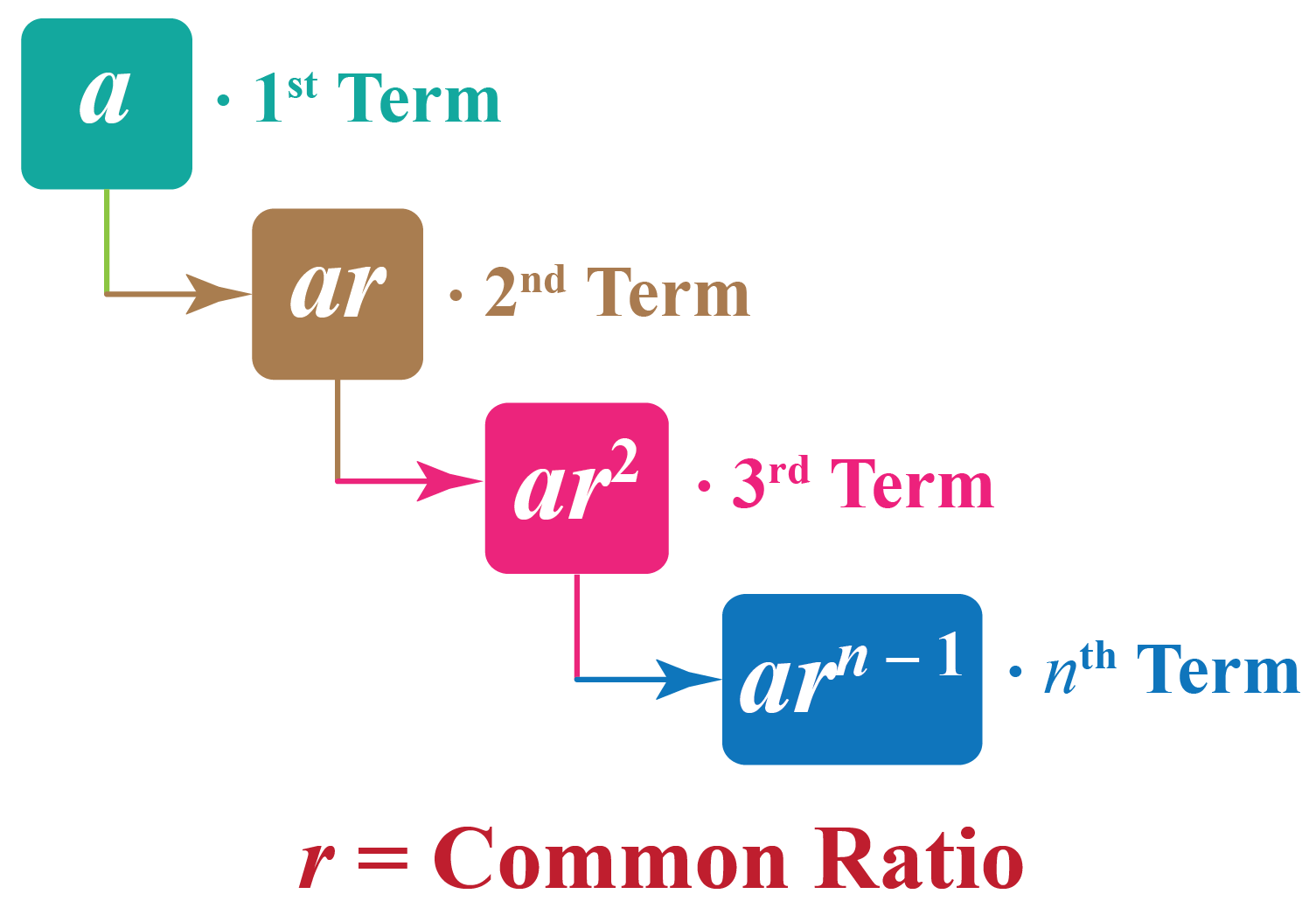### General Notations

We generally use the notation $$a_{n}$$ to represent the $$n^{\text{th}}$$ term in an arithmetic progression or a geometric progression.

The common difference is denoted by $$d$$ and the common ratio is denoted by $$r$$.

## General Form and Nth Terms

Let's learn the general forms for arithmetic progression and geometric progression.

### Arithmetic Progression: Formula

Let the first term of the sequence be $$a$$ and the common difference be $$d$$.

Then, $$n^{\text{th}}$$ term of the sequence is given by:

 \begin{align}a_{n}=a+(n-1)d\end{align}

The nth term is the arithmetic sequence explicit formula and can be useful to find any term of the progression.

Experiment with the simulation below to determine the $$n^{\text{th}}$$ term of different arithmetic progressions.

Change the sliders for $$a$$ and $$d$$ to get different sequences and change the slider for $$n$$ to get the number of terms of that sequence.

### Geometric Progression: Formula

Let the first term of the sequence be $$a$$ and the common ratio be $$r$$.

Then, $$n^{\text{th}}$$ term of the sequence is given by:

 \begin{align}a_{n}=ar^{n-1}\end{align}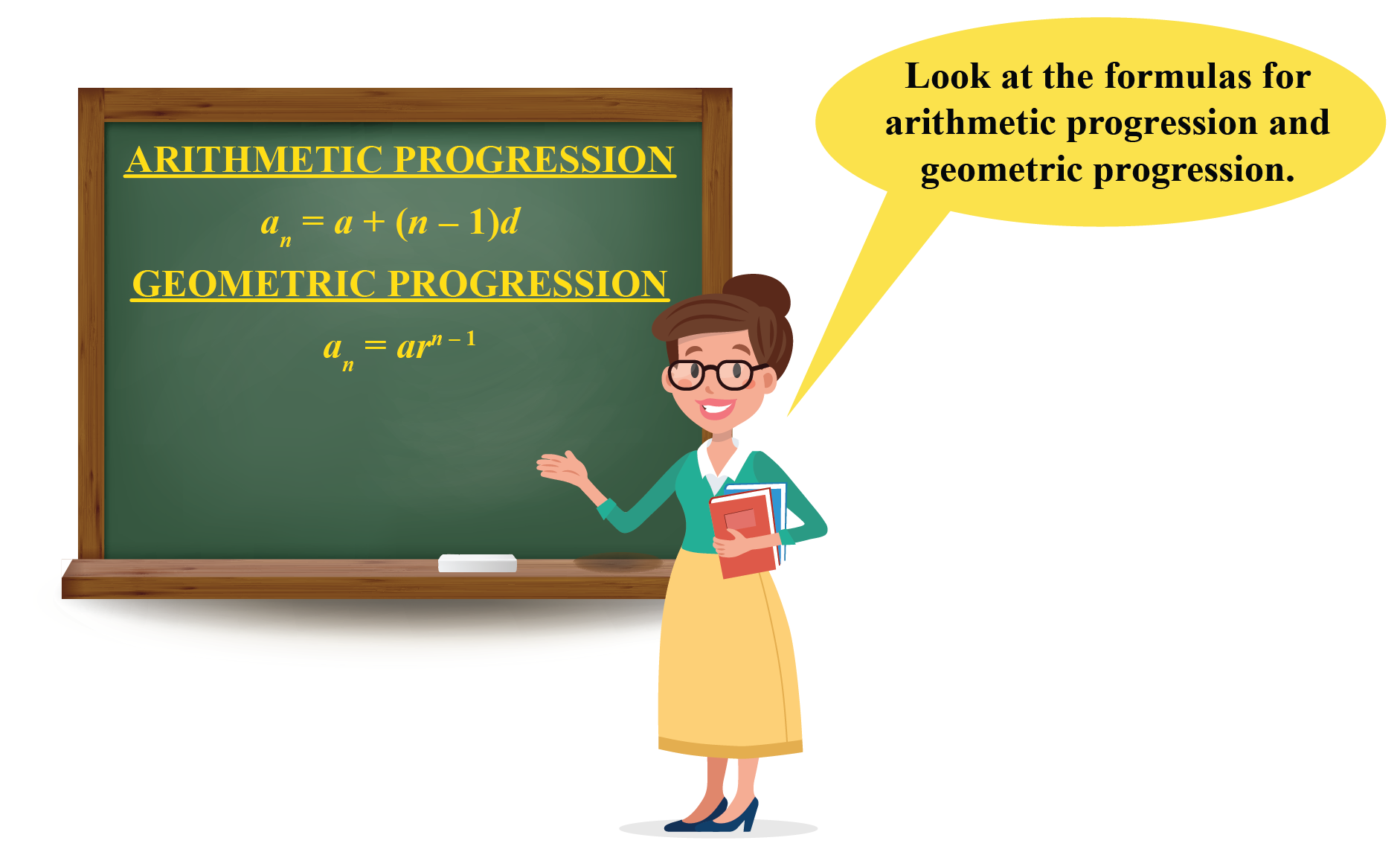Sum Of N Terms

### Sum Of Arithmetic Progression

Consider an arithmetic progression whose first term is $$a$$ and the common difference is $$d$$.

The sum of the first $$n$$ terms of an arithmetic progression is:

 $S_n=\frac{n}{2}(2 a+(n-1) d)$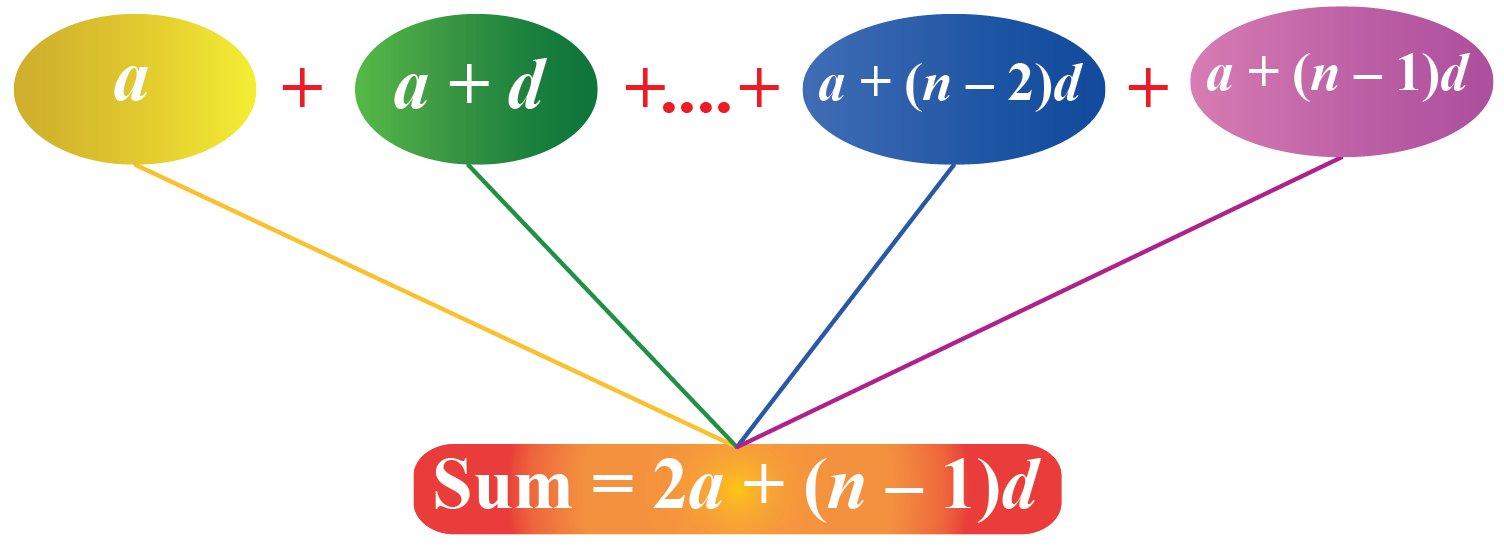Now, we already know that $$n^{\text{th}}$$ term of an arithmetic progression is \begin{align}a_{n}=a+(n-1)d\end{align}.

So, the sum formula of an arithmetic progression can be written as

\begin{align}S_n&=\frac{n}{2}(2 a+(n-1) d)\\&=\frac{n}{2}(a+a+(n-1) d)\\&=\frac{n}{2}(a+a_n)\end{align}

So, another formula to find the sum of the first $$n$$ terms of an arithmetic progression is:

 $S_n=\frac{n}{2}(a+a_n)$

### Geometric Progression: Sum

Consider a geometric progression whose first term is $$a$$ and the common ratio is $$r$$.

The sum of the first $$n$$ terms of a geometric progression is:

 $S_n=\dfrac{a(1-r^n)}{1-r}$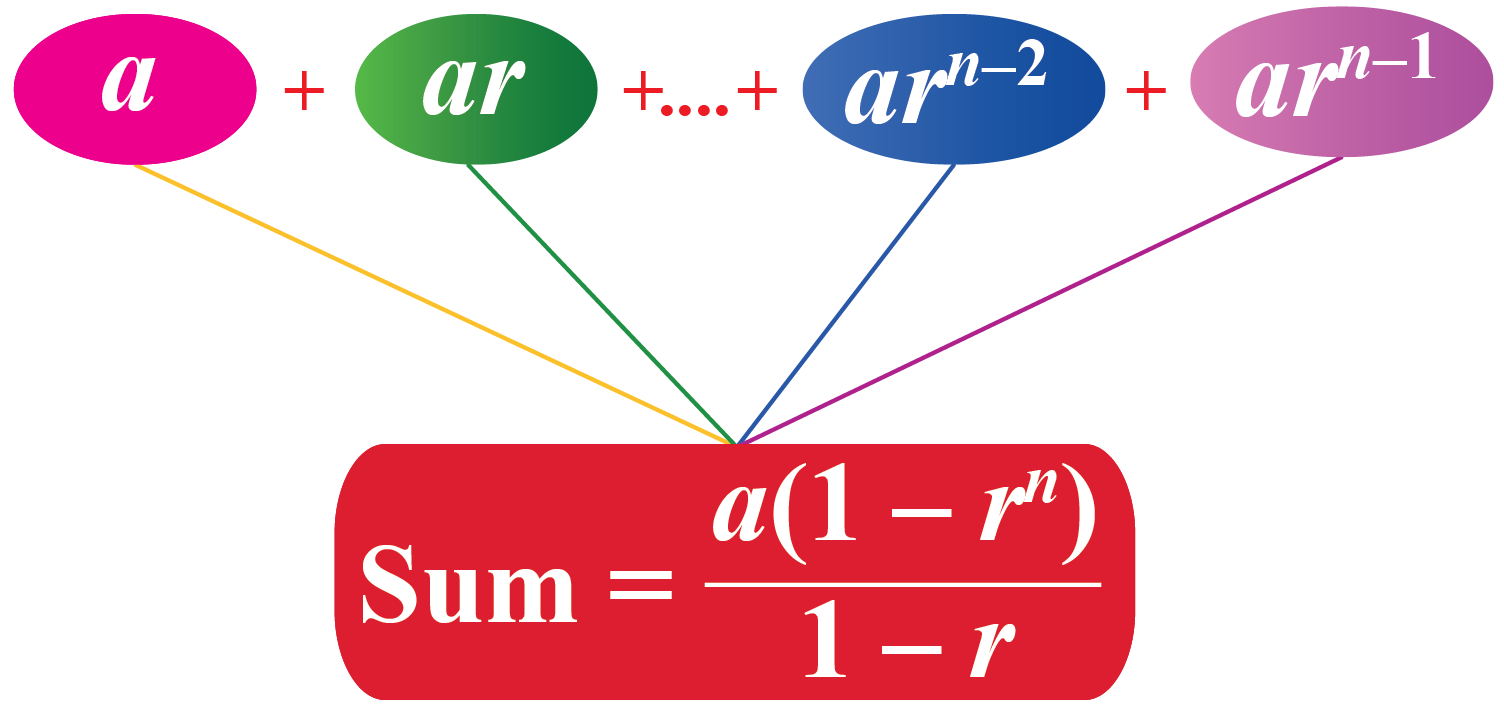## Solved Examples

 Example 1

A taxi charges $2 for the first mile and$1.5 for each subsequent mile.How much Katie needs to pay to the taxi driver if she travels 5 miles?

Solution

The taxi fare for the first few miles are $2,$3.5, 5, ... It forms an arithmetic progression with a common difference, $$d=1.5$$ and first term, $$a=2$$ The 5th term of the sequence will give the taxi fare for the first 5 miles. The 5th term of the sequence will be given by $$a+4d$$. \begin{align}a+4d&=2+4(1.5)\\&=2+6\\&=8\end{align}  $$\therefore$$ Katie pays $$8$$ to the taxi driver for 5 miles.  Example 2 Mushi put30 in her piggy bank when she was 7 years old.

She increased the amount on her each successive birthday by $3.How much money will she have on her 12th birthday? Solution The amount in Mushi's piggy bank follows the pattern of$30, $33,$36, and so on.

The succeeding terms are obtained by adding a fixed number, that is, 3 This fixed number is a common difference. The total money collected on her 12th birthday will be the sum of the first 6 terms on this arithmetic progression. \begin{align}S_n&=\frac{n}{2}(2 a+(n-1) d)\\S_{6}&=\frac{6}{2}(60+(6-1)3)\\&=3\times 75\\&=225\end{align}  $$\therefore$$ Mushi will have225 in her piggy bank on her 12th birthday.
 Example 3

Jack is a fitness freak.

He started practicing by doing 10 pushups on his first day.Every day, he did 5 pushups extra than the previous day.

How many pushups did he do on the last day of the first week?

### Solution

The number of pushups represents the arithmetic progression given by 10, 15, 20, and so on.

The last day of the week is the 7th term of this sequence.

The 7th term of the sequence will be given by $$a+6d$$.

\begin{align}a+6d&=10+6(5)\\&=40\end{align}

 $$\therefore$$ Jack did 40 pushups on the last day of the week.

 Example 4

Look at the pattern shown below.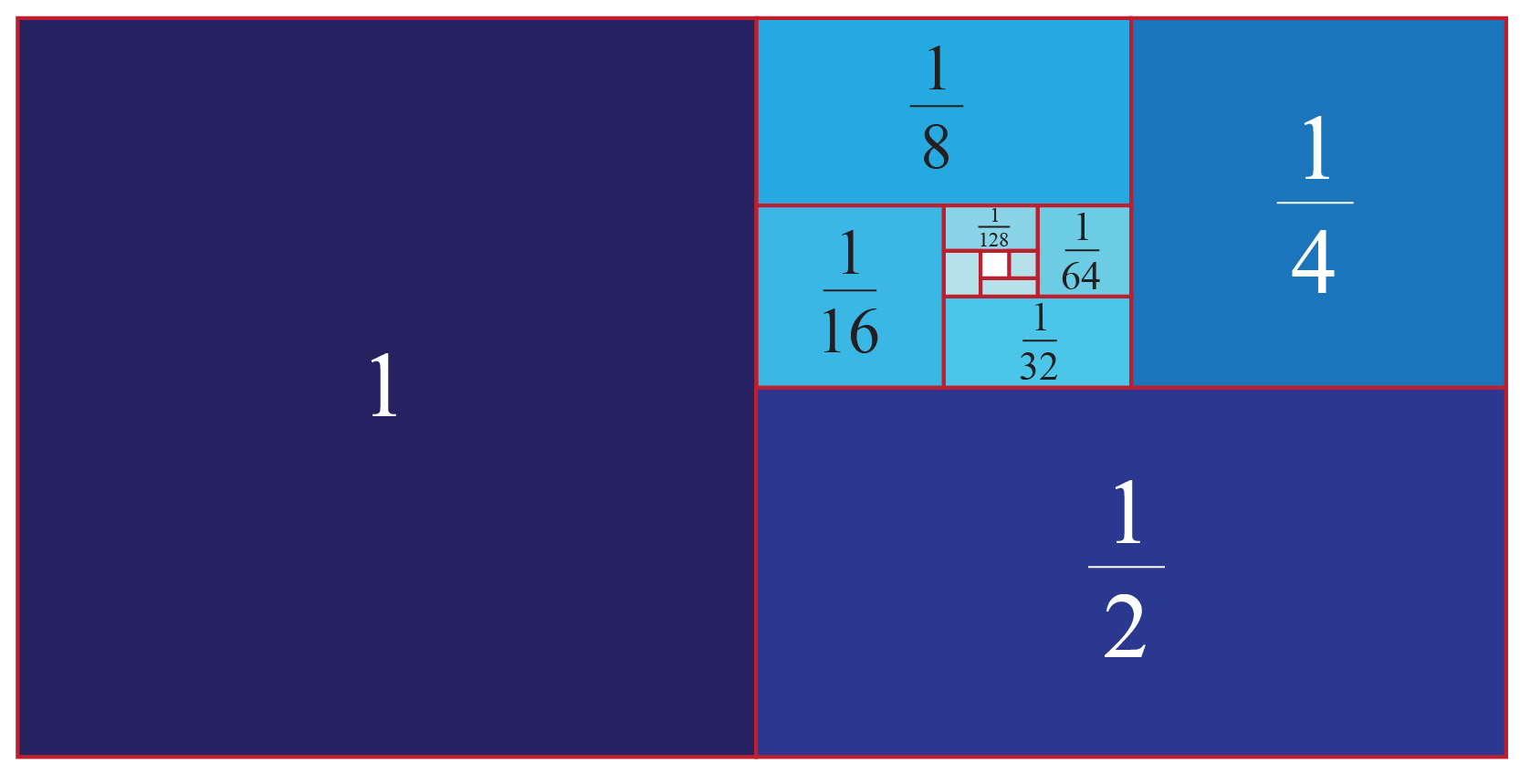Observe that each square is half of the size of the square next to it.

Which sequence does this pattern represent?

### Solution

Let's write the sequence represented in the figure.

$1, \dfrac{1}{2}, \dfrac{1}{4}, \dfrac{1}{8}, \dfrac{1}{16},...$

Every successive term is obtained by dividing its preceding term by 2

The sequence exhibits a common ratio of $$\dfrac{1}{2}$$

 $$\therefore$$ The pattern represents the geometric progression.
 Example 5

Hailey's teacher asks her to find the $$10^{\text{th}}$$ term of the sequence: 1, 4, 16, 64, ...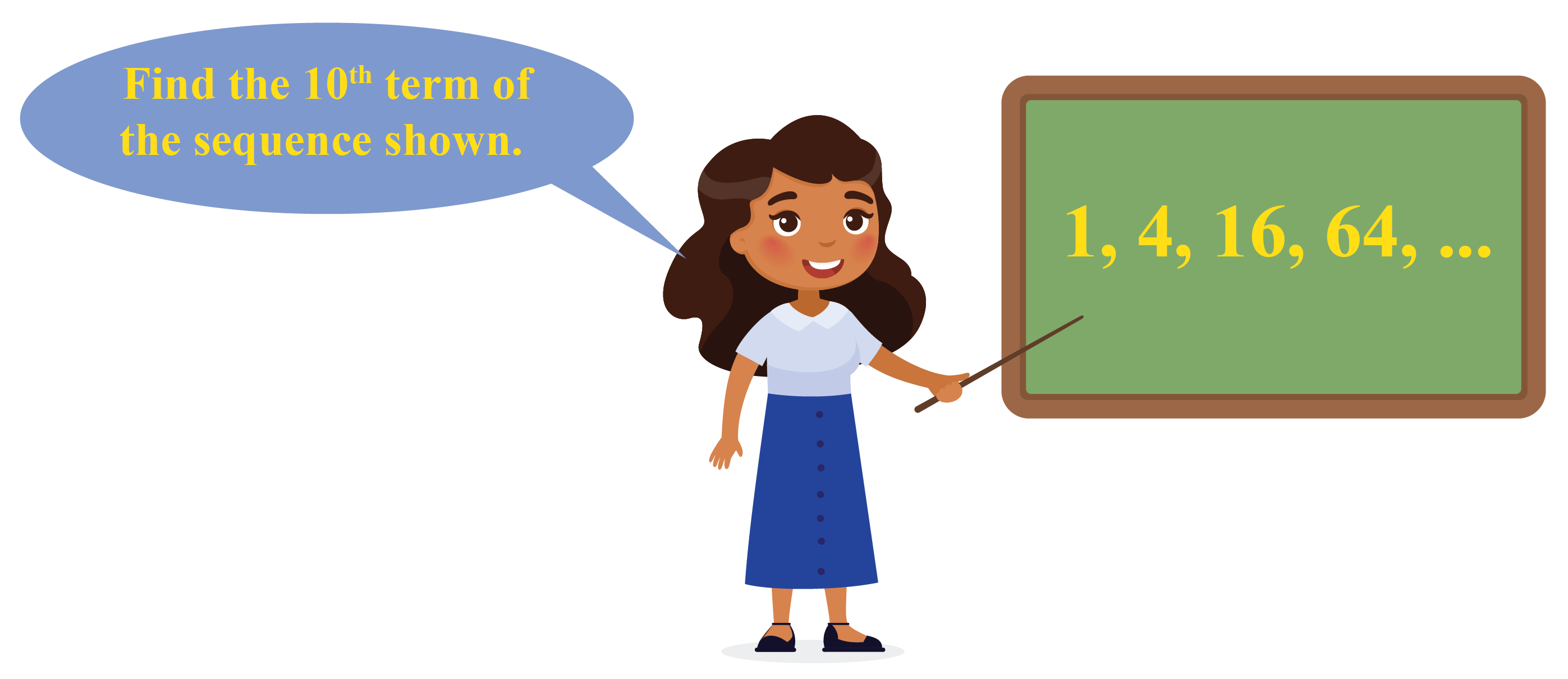Can you help her?

Solution

Observe that $$\dfrac{4}{1}=\dfrac{16}{4}=\dfrac{64}{16}=4$$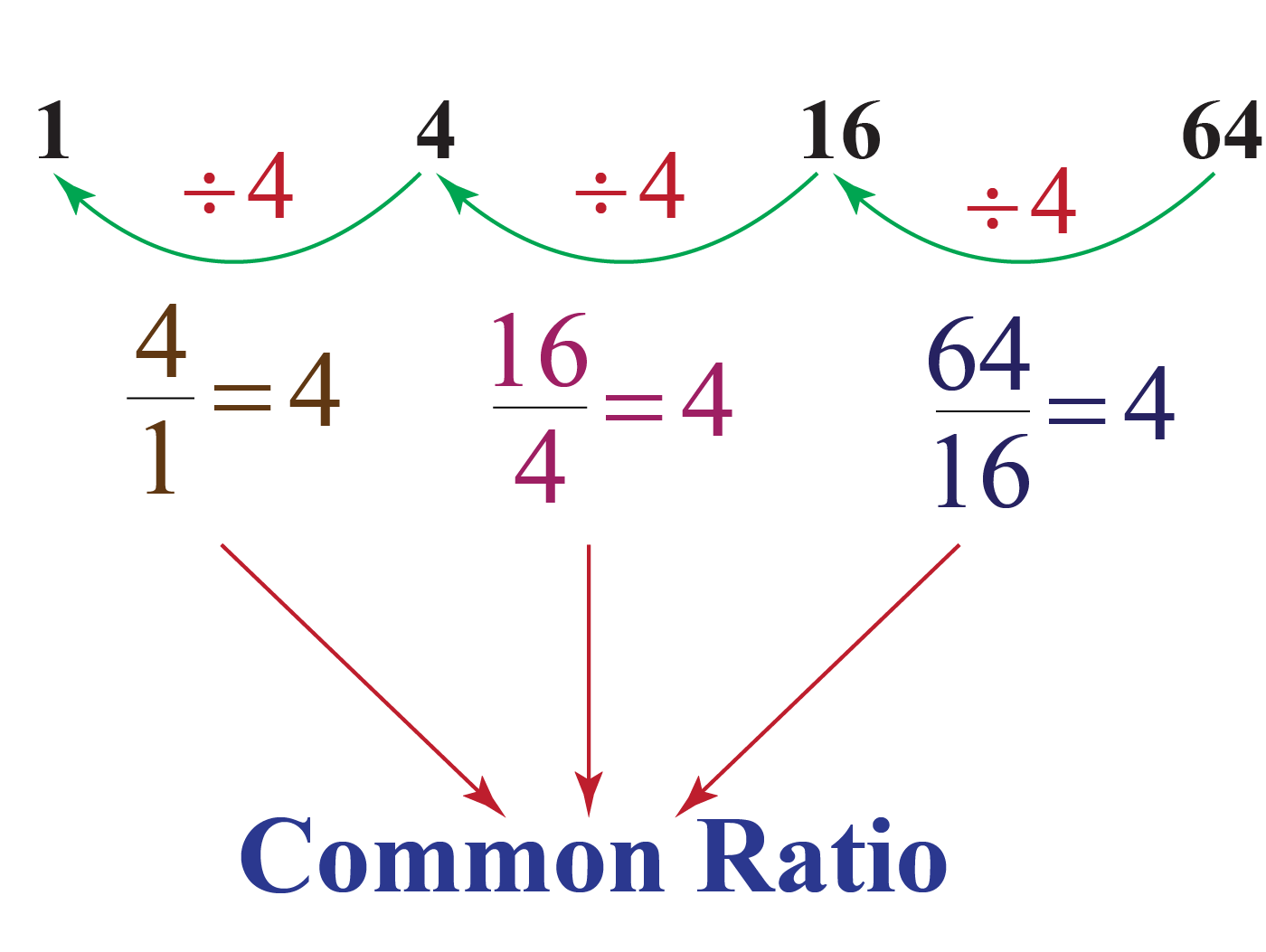Here, $$r=4$$ is a common ratio.

So, the given sequence represents the geometric progression.

The 10th term of the sequence will be given by $$ar^{9}$$.

\begin{align}ar^{9}&=1\times 4^9\\&=4^9\end{align}

 $$\therefore$$ The 10th term of the sequence is $$4^9$$.
 Example 6

A person has 2 parents, 4 grandparents, 8 great-grandparents, and so on.Can you determine the number of ancestors during the 8 generations preceding his own?

Solution

The number of ancestors in each generation represents the term of a geometric progression.

So, $$a=2$$, $$r=2$$, and $$n=10$$.

We will use the geometric progression sum formula.

\begin{align}S_n&=\dfrac{a(1-r^n)}{1-r}\\S_{10}&=\dfrac{2\left(1-2^{10}\right)}{1-2}\\&=2(2^{10}-1)\\&=2046\end{align}

 $$\therefore$$ The required number of ancestors is 2046.Think Tank
 1. The summer Olympics are held after every 4 years.Can you think of any formula for arithmetic progression to represent the years of the summer Olympics starting from 2012?

## Interactive Questions

Here are a few activities for you to practice.

## Let's Summarize

We hope you enjoyed learning about sequences with the examples and practice questions. Now, you will be able to easily remember the formulas of sequence and solve problems on sequences in math, which include arithmetic sequence, geometric sequence, harmonic sequence, and other types of sequences.

At Cuemath, our team of math experts is dedicated to making learning fun for our favorite readers, the students!

Through an interactive and engaging learning-teaching-learning approach, the teachers explore all angles of a topic.

Be it worksheets, online classes, doubt sessions, or any other form of relation, it’s the logical thinking and smart learning approach that we, at Cuemath, believe in.

## 1. What is a geometric progression in mathematics?

A geometric progression is a sequence where every term bears a constant ratio to its preceding term.

## 2. How do you know if a sequence is arithmetic or geometric?

If each successive term of a sequence is less than the preceding term by a fixed number, then the sequence is arithmetic.

If each successive term of a sequence is a product of the preceding term and a fixed number, then the sequence is geometric.

## 3. What is the nth term of a geometric sequence?

The nth term of a geometric sequence with first term $$a$$ and the common ratio $$r$$ is given by $$a_{n}=ar^{n-1}$$.

## 4. What is the nth term of an arithmetic sequence?

The nth term of an arithmetic sequence with first term $$a$$ and the common difference $$d$$ is given by $$a_{n}=a+(n-1)d$$.

## 5. What is an arithmetic progression in mathematics?

An arithmetic progression is a sequence of numbers in which each successive term is a sum of its preceding term and a fixed number.

## 6. How to find the sum of arithmetic progression?

The sum of arithmetic progression with first term $$a$$ and the common difference $$d$$ is given by following formulas:

1. $$S_n=\frac{n}{2}(2 a+(n-1) d)$$
2. $$S_n=\frac{n}{2}(a+a_{n})$$

## 7. How to find the sum of geometric progression?

The sum of geometric progression with first term $$a$$ and the common ratio $$r$$ is given by $$S_n=\dfrac{a(1-r^n)}{1-r}$$.

Progressions
grade 10 | Questions Set 1
Progressions
Progressions
grade 10 | Questions Set 2
Progressions
More Important Topics
Numbers
Algebra
Geometry
Measurement
Money
Data
Trigonometry
Calculus
More Important Topics
Numbers
Algebra
Geometry
Measurement
Money
Data
Trigonometry
Calculus